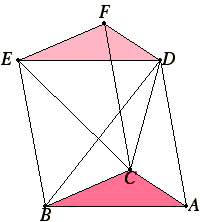# Proposition 7

Any prism with a triangular base is divided into three pyramids equal to one another with triangular bases.

Let there be a prism with the triangular base ABC and DEF opposite.

I say that the prism ABCDEF is divided into three pyramids equal to one another, which have triangular bases.Join BD, EC, and CD.

Since ABED is a parallelogram, and BD is its diameter, therefore the triangle ABD equals the triangle EBD. Therefore the pyramid with triangular base ABD and vertex C equals the pyramid with triangular base DEB and vertex C.

But the pyramid with triangular base DEB and vertex C is identical with the pyramid with triangular base EBC and vertex D, for they are contained by the same planes.

Therefore the pyramid with triangular base ABD vertex C is also equal to the pyramid with triangular base EBC and vertex D.

I.34

Again, since FCBE is a parallelogram, and CE is its diameter, therefore the triangle CEF equals the triangle CBE.

XII.5

Therefore the pyramid with triangular base BCE and vertex D equals the pyramid with triangular base ECF and vertex D.

But the pyramid with triangular base BCE and vertex D was proved equal to the pyramid with triangular base ABD and vertex C, therefore the pyramid with triangular base CEF and vertex D equals the pyramid with triangular base ABD and vertex C. Therefore the prism ABCDEF is divided into three pyramids equal to one another which have triangular bases.

And, since the pyramid with triangular base ABD and vertex C is identical with the pyramid with triangular base CAB and vertex D, for they are contained by the same planes, while the pyramid with triangular base ABD vertex C was proved to be a third of the prism with triangular base ABC and DEF opposite, therefore the pyramid with triangular base ABC and vertex D is a third of the prism with the same base ABC, and DEF opposite.

Therefore, any prism with a triangular base is divided into three pyramids equal to one another with triangular bases.

Q.E.D.

# Corollary

From this it is clear that any pyramid is a third part of the prism with the same base and equal height.

## Guide

The proof of this proposition is easier than it looks. The triangles ABD and EBD are equal since each is half the parallelogram ABED. Now since the pyramids ABDC and DEBC have equal bases and the same altitude, by XII.5, they are equal pyramids. A similar argument shows pyramids BCEDand ECFD are equal. But DEBC and BCED are the same pyramid named differently. So the prism is divided into three equal pyramids.

#### Use of this proposition

This proposition is used in the next two propositions about volumes of pyramids and in XII.10 following them about volumes of cones and cylinders.